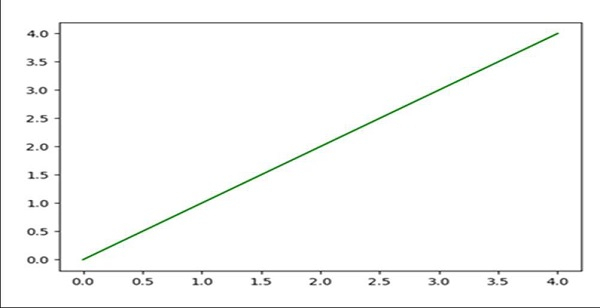# How do I get interactive plots again in Spyder/Ipython/matplotlib?

To get interactive plots, we need to activate the figure. Using plt.ioff() and plt.ion(), we can perform interactive actions with plot.

## Steps

• Create fig and ax variables using subplots method, where default nrows and ncols are 1.

• Draw a line, using plot() method.

• Set the color of the line, i.e., orange.

• Stopped the interaction, using plt.ioff() method.

• To make the interaction plots, change the color of the line coordinate.

• Start the interaction, using plt.ion() method.

## Example

To use interactive plot in Ipython -

In : %matplotlib auto

Using matplotlib backend: GTK3Agg

In : import matplotlib.pyplot as plt

In : fig, ax = plt.subplots()    # Diagram will get popped up, Let’s interact.

In : ln, = ax.plot(range(5))     # Drawing a line

In : ln.set_color('orange')      # Changing drawn line to orange

In : plt.ioff()                  # Stopped interaction

In : ln.set_color('green')
# Since we have stopped the interaction in the last step

In : plt.ion()                   # Started interaction

## Output